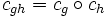# Group acts as automorphisms by conjugation

This article gives the statement, and possibly proof, of a basic fact in group theory.
View a complete list of basic facts in group theory
VIEW FACTS USING THIS: directly | directly or indirectly, upto two steps | directly or indirectly, upto three steps|
This article describes a pivotal group action: a group action on a set closely associated with the group. This action is important to understand and remember.
View other pivotal group actions

## Statement

Let$G$ be a group. For any$g \in G$, define the map:$c_g:G \to G$

given by:$c_g(x) := gxg^{-1}$

(this is termed conjugation by$g$ or the inner automorphism induced by$g$).

Then, the following are true:

• For every$g \in G$,$c_g$ is an automorphism of$G$ (an automorphism arising this way is termed an inner automorphism).
• The map from$G$ to its automorphism group$\operatorname{Aut}(G)$ that sends an element$g$ to the automorphism$c_g$, is a homomorphism of groups.

## Proof

### Proof that every conjugation is an automorphism

Given: A group$G$, an element$g \in G$

To prove: The map$c_g: x \mapsto gxg^{-1}$ is an automorphism of$G$

Proof:$c_g$ is a map from$G$ to$G$. We need to thus prove three things:

•$c_g(xy) = c_g(x)c_g(y)$

The key idea for this is the fact that$g^{-1}g = e$. Formally:$c_g(x)c_g(y) = (gxg^{-1})(gyg^{-1}) = gx(g^{-1}g)yg^{-1} = gxeyg^{-1} = gxyg^{-1} = c_g(xy)$

•$c_g(e) = e$

The key idea for this is the fact that$gg^{-1} = e$. Formally:$c_g(e) = geg^{-1} = gg^{-1} = e$

•$c_g(x^{-1}) = c_g(x)^{-1}$

Here, we use the fact that the inverse of a product of elements is the product of their inverses, in reverse order (i.e. the inverse map is involutive). Thus, we have:$c_g(x)^{-1} = (gxg^{-1})^{-1} = (g^{-1})^{-1}x^{-1} g^{-1} = gx^{-1}g^{-1} = c_g(x^{-1})$

Note that for the proof to go through we need to use the fact that$g$ and$g^{-1}$ are left and right inverses of each other.

NOTE: It actually suffices to prove only the first of these three things, because to test whether a map between groups is a homomorphism of groups, it suffices to check whether it sends products to products. However, when working in somewhat greater generality than groups, it becomes important to check the other conditions, and they're explained here for illustrative purposes.

Thus, every$c_g$ is a homomorphism. It remains to show that this homomorphism is injective and surjective. Injectivity is clear, because$c_g(x) = e \implies x = e$. For surjectivity, note that given any$y \in G$, setting:$x := g^{-1}yg$

yields$c_g(x) = y$. (This also becomes clearer from the fact that the map$g \mapsto c_g$ is itself a homomorphism of groups).

Thus,$c_g$ is an automorphism.

### Proof that the map is a homomorphism

Given: A group$G$

To prove: The map$g \mapsto c_g$, is a homomorphism from$G$ to$\operatorname{Aut}(G)$

Proof: We'll check three things:

•$c_{gh} = c_g \circ c_h$

The proof again uses the reversal law for inverses (inverse map is involutive):$c_{gh}(x) = ghx(gh)^{-1} = ghxh^{-1}g^{-1} = g(c_h(x))g^{-1} = c_g(c_h(x)) = (c_g \circ c_h)(x)$

•$c_e$ is the identity map:$c_e(x) = exe^{-1} = x$

•$c_g^{-1} = c_{g^{-1}}$

This follows from the first two facts, because$c_g \circ c_{g^{-1}} = c_{gg^{-1}} = c_e$ which is the identity map, and the same holds for composition in the other order.